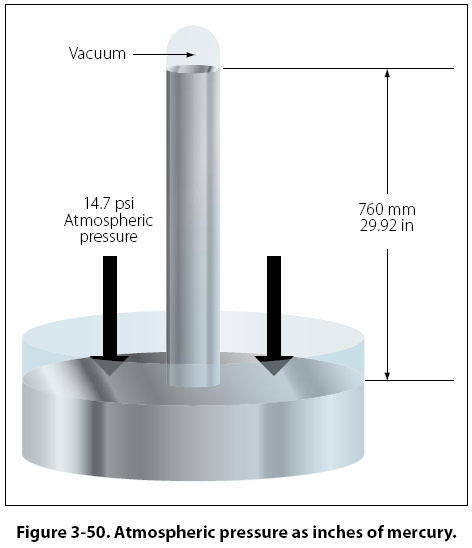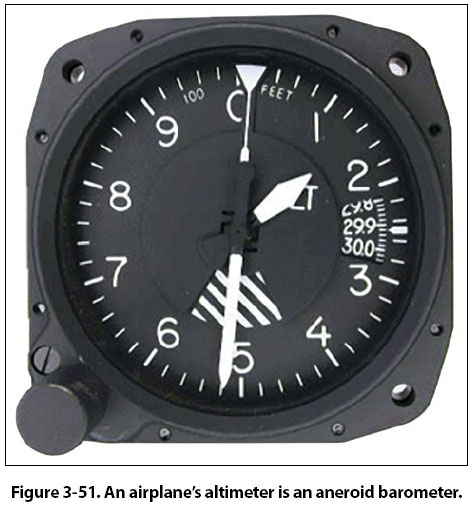Atmospheric Pressure The human body is under pressure, since it exists at the bottom of a sea of air. This pressure is due to the weight of the atmosphere. On a standard day at sea level, if a 1-in2 column of air extending to the top of the atmosphere was weighed, it would weigh 14.7 lb. That is why standard day atmospheric pressure is said to be 14.7 pounds per square inch (14.7 psi). Since atmospheric pressure at any altitude is due to the weight of air above it, pressure decreases with increased altitude. Obviously, the total weight of air above an area at 15,000 ft would be less than the total weight of the air above an area at 10,000 ft. Atmospheric pressure is often measured by a mercury barometer. A glass tube somewhat over 30 inches in length is sealed at one end and then filled with mercury. It is then inverted and the open end placed in a dish of mercury. Immediately, the mercury level in the inverted tube will drop a short distance, leaving a small volume of mercury vapor at nearly zero absolute pressure in the tube just above the top of the liquid mercury column. Gravity acting on the mercury in the tube will try to make the mercury run out. Atmospheric pressure pushing down on the mercury in the open container tries to make the mercury stay in the tube. At some point these two forces (gravity and atmospheric pressure) will equilibrate and the mercury will stabilize at a certain height in the tube. Under standard day atmospheric conditions, the air in a 1-in2 column extending to the top of the atmosphere would weigh 14.7 lb. A 1-in2 column of mercury, 29.92 inches tall, would also weigh 14.7 lb. That is why 14.7 psi is equal to 29.92 "Hg. Figure 3-50 demonstrates this point.A second means of measuring atmospheric pressure is with an aneroid barometer. This mechanical instrument is a much better choice than a mercury barometer for use on airplanes. Aneroid barometers (altimeters) are used to indicate altitude in flight. The calibrations are made in thousands of feet rather than in psi or inches of mercury. For example, the standard pressure at sea level is 29.92 "Hg, or 14.7 psi. At 10,000 feet above sea level, standard pressure is 20.58 "Hg, or 10.10 psi. Altimeters are calibrated so that if the pressure exerted by the atmosphere is 10.10 psi, the altimeter will point to 10,000 ft. [Figure 3-51]©AvStop Online Magazine                                                                                                                                                      Contact Us              Return To Books